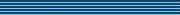| home      | people     | research     | publications    | seminars    | events     | contactCommunication Networks   | Systems Biology   | Hybrid Systems    | Machine Learning   | Dynamics & Interaction### Schools Mathematics Grand Challenge

Week ten's Puzzles

Problem 28:

The twenty eighth problem was:

A new train timetable is being designed for the route between Maynooth and Dublin, and it is hoped to greatly increase the frequency of trains on the line. However, the timetable for departures from Maynooth must satisfy certain conditions.

1. The first train to leave Maynooth in the morning must depart at 7.00am exactly; 2. The gap between successive departures from Maynooth must be either exactly 5 minutes or exactly 17 minutes.

Working with these rules, what is the latest time before 12 noon at which no train can possibly leave Maynooth for Dublin? For example, no train can possibly leave at 7.11 or at 7.06.

The solution was:

This is a more complicated version of fizz buzz bang. Let's look at the train times we can get. If we don't use any 17 minute gaps, the trains will leave at 7.00, 7.05, 7.10, 7.15, 7.20, 7.25, 7.30...
Suppose the answer is X minutes after 7.00am - we know now X does not end in a 5 or a 0.

If we use exactly one 17 minute gap (we might as well use it as early as possible), the trains will leave at 7.17, 7.22, 7.27, 7.32, 7.37...
Now we know that if X is > 16, X does not end in a 2 or a 7.

Two 17 minute gaps will tell us that if X is >33, X does not end in a 4 or a 9.
Three 17 minute gaps will tell us that if X is >50, X does not end in a 1 or a 6.
Four 17 minute gaps will tell us that if X is >67, X does not end in an 8 or a 3.

What do we know? X < 68 and does not end in a 0,1,2,4,6,7,9. The biggest such number is 63. 63 minutes after 7.00am is 8.03am.

Problem 29:

The twenty ninth problem was:

How many positive whole numbers have the following properties:

1. No digit of the number is 0;
2. The sum of the digits of the number is equal to 9;
3. The number has an even number of even digits?

The solution was:

We might guess (correctly) that exactly half of the positive whole numbers from last week have an even number of even digits (the other half have an odd number of even digits). This tells us the answer is 128.

In fact 128 is small enough that just writing all the matching positive whole numbers out is a quick way to do this problem.

More cleverly, suppose that to begin with the first digit is one. Now you have to choose whether to (A) add one to the first (=last) digit or whether to (B) make a new last digit of 1. After you make that choice, you will then have to choose whether to (A) add one to the last digit of whatever you currently have or (B) to make a new last digit of 1. Repeat this until the sum of the digits is 8.
For example
1
choose (B)
11
choose (A)
12
choose (B)
121
choose (A)
122
choose (A)
123
choose (A)
124
choose (B)
1241
When you have the sum of digits being 8, you will not have a choice any more as you will be forced to choose A or B depending on how many even digits you currently have. In our example we have 2 even digits which is even, so we must choose B and get 12411.

We had to choose A or B seven times so the total number of ways is is 2*2*2*2*2*2*2=2^7=128

Problem 30:

The thirtieth problem was:

John is holding a party at his house and has bought one very large pizza. He wishes to share it out by making a number of straight line cuts across the pizza such as that shown below (Each cut must go from one point on the circumference of the pizza to a different point on its circumference).

If John makes 12 cuts through the pizza, and nobody takes more than one piece of pizza, what is the maximum number of people that can get a piece?The solution was:

Again the numbers are small enough here that drawing a huge circle and counting is a reasonable way to solve this problem. Note that three lines should never meet exactly (if they do there's a possible extra piece in there!)

Every new cut gives us one extra piece automatically, and one extra piece for every cut that the new cut crosses.

This means that the maximum number of pieces for 12 cuts is the maximum number of pieces for 11 cuts + 12. So we can generate the following table

 Number of cuts 0 1 2 3 4 5 6 Maximum number of pieces 1 1+1=2 2+2=4 4+3=7 7+4=11 11+5=16 16+6=22 Number of cuts 7 8 9 10 11 12 Maximum number of pieces 22+7=29 29+8=37 37+9=46 46+10=56 56+11=67 67+12=79

You could solve this pattern to see that in general the maximum number of pieces for n cuts is 1+.5*n*(n+1) or 1+n+(n choose 2).